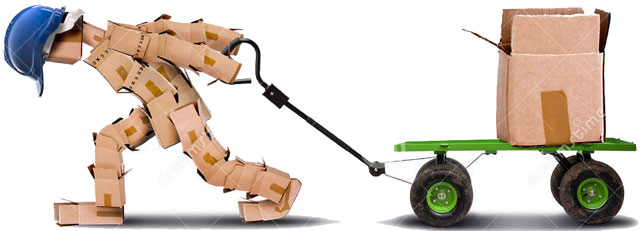You might think about work as, reading books and doing homework. You may have also heard the sentence “hard work leads to success”. But, in physics work has its own meaning and it is different from the previously described example.

## What is Work?In physics, work is done when a force is applied to an object and it travels some distance. For example, you kicked a ball with a certain amount of force and ball traveled few meters, then you had done a certain amount of work on the ball.

## How is Work measured?

Work is the scalar quantity, means it doesn’t need direction to define it. Work is measured in joule or newton-meter. This is the same unit in which energy is measured. The work done on an object is found by multiplying the force exerted on an object with the distance that it traveled.

Work = Force*distance

But, there are also some complications in calculating the work which may sometimes confuse you. Here are the few things to remember when calculating the work.

1. If you are applying a force on an object but it is not moving, then work will be zero. Because object didn’t travel any distance. For example, you are pushing a wall with all of your force, but the wall is not moving. So, your work done will be zero.
2. If you are applying a force on an object and it is moving in a path that brings it back from where it started. Then, work done on that object is also zero. Because the net distance traveled by the object is zero.
For example, a race car is starting from a starting point and traveling in a circular path and it comes back again at the same starting point. Then, work done by the car is zero because it didn’t travel any net distance.

Example: A man is pushing a box with a force 50 newtons. The box has traveled the distance of 100 meters. Calculate the work done by the man.

Solution:

Applied Force = 50 N

Distance covered = 100 meters

Work = Force*distance = 50*100 = 5000 joules or N-m

## Facts

• If a force is applied in opposite direction to the covered distance, then the work is said to be negative work.
• An object that falls from a height also performs work due to gravitational force and height.
• In space when an object is accelerated to a certain speed then the force is removed from it. But, the object doesn’t lose its speed because there is no any air friction in space. So, work done by objects flying in space is also zero.